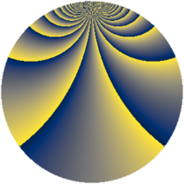# Properties

 Label 1078.2.baLevel $1078$ Weight $2$ Character orbit 1078.ba Rep. character $\chi_{1078}(13,\cdot)$ Character field $\Q(\zeta_{70})$ Dimension $1344$ Sturm bound $336$

# Related objects

## Defining parameters

 Level: $$N$$ $$=$$ $$1078 = 2 \cdot 7^{2} \cdot 11$$ Weight: $$k$$ $$=$$ $$2$$ Character orbit: $$[\chi]$$ $$=$$ 1078.ba (of order $$70$$ and degree $$24$$) Character conductor: $$\operatorname{cond}(\chi)$$ $$=$$ $$539$$ Character field: $$\Q(\zeta_{70})$$ Sturm bound: $$336$$

## Dimensions

The following table gives the dimensions of various subspaces of $$M_{2}(1078, [\chi])$$.

Total New Old
Modular forms 4128 1344 2784
Cusp forms 3936 1344 2592
Eisenstein series 192 0 192

## Trace form

 $$1344 q - 56 q^{4} - 10 q^{7} - 56 q^{9} + O(q^{10})$$ $$1344 q - 56 q^{4} - 10 q^{7} - 56 q^{9} - 6 q^{11} - 2 q^{14} - 30 q^{15} + 56 q^{16} - 70 q^{17} - 28 q^{20} - 14 q^{22} - 52 q^{23} - 56 q^{25} - 28 q^{26} + 126 q^{27} + 10 q^{28} + 20 q^{29} + 60 q^{35} + 56 q^{36} - 16 q^{37} - 28 q^{38} - 70 q^{40} + 126 q^{42} + 26 q^{44} - 112 q^{45} - 154 q^{47} - 112 q^{49} - 200 q^{51} + 152 q^{53} + 168 q^{55} + 20 q^{56} - 80 q^{57} - 140 q^{58} + 28 q^{59} - 20 q^{60} - 70 q^{62} - 40 q^{63} - 56 q^{64} + 32 q^{67} + 56 q^{69} + 26 q^{70} - 72 q^{71} - 40 q^{72} - 210 q^{73} - 40 q^{74} + 46 q^{77} + 32 q^{78} - 60 q^{79} - 100 q^{81} - 20 q^{84} + 100 q^{85} + 90 q^{86} + 36 q^{88} + 168 q^{89} - 184 q^{91} + 28 q^{92} - 84 q^{93} + 350 q^{94} - 20 q^{95} - 816 q^{99} + O(q^{100})$$

## Decomposition of $$S_{2}^{\mathrm{new}}(1078, [\chi])$$ into newform subspaces

The newforms in this space have not yet been added to the LMFDB.

## Decomposition of $$S_{2}^{\mathrm{old}}(1078, [\chi])$$ into lower level spaces

$$S_{2}^{\mathrm{old}}(1078, [\chi]) \cong$$ $$S_{2}^{\mathrm{new}}(539, [\chi])$$$$^{\oplus 2}$$